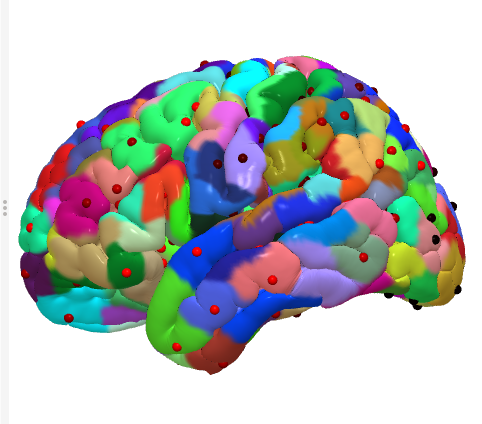# Suggestions on solving a problem with SurfDist

Hello,

I am currently using SurfDist to solve a problem that involves the geodesic distance between node pairs on the surface mesh. I know this is a computationally expensive process, especially if I am calculating the distances between many pairs of nodes. Perhaps, you can provide me with some insight on how to approach this problem using the fastest method. Here is what I would like to do:

Problem #1: I have a set of 400 ROIs (based on the Schaefer parcellation) on the standard 141 ld mesh surface. Given node n in parcel p, I would like to find the minimum geodesic distance between node n and all parcels != p within the same hemisphere as p.

Theoretically, this only require 199 pairs of nodes to calculate all of the geodesic distances. However, one does not know ahead of time which node within each ROI has the closest geodesic distance to node n. Therefore, to be most thorough, I think I would need to calculate the geodesic distance between node n and every node at the perimeter of every parcel within the same hemisphere. So, as a first question, how could I get the set of all “perimeter” nodes for every ROI within a hemisphere? And if you see a faster way of solving this, then let me know.

Problem #2: Imagine the same set of ROIs and surface as before. This time I want to find the set of all nodes that are less than a certain geodesic distance (e.g. 100mm) away from node n. This is perhaps more computationally difficult because I want to find all of the nodes (not parcels) that are a certain distance away from node n. But, there may be a way to speed up this search by eliminating all nodes that are clearly too far away from node n because they have a Euclidean distance greater than 100mm. Euclidean distance is much faster to find than geodesic distance, but still I imagine finding the Euclidean distance between ~198k pairs of nodes to threshold for the pairs of nodes that need geodesic distance calculated would take a long time. Another way to approach the problem would be to check all of the Euclidean distances or geodesic distances from n to nodes in adjacent parcels spreading radially outward from parcel p that contains n. If I still find Euclidean distances less than 100mm, then I could expand my search to the next set of neighboring parcels, but otherwise, I don’t need to search any farther. I have no idea how I would begin to do this though, especially because I don’t know how to find the parcels that are adjacent to a certain parcel. Let me know if you have any thoughts on this second problem!

Thanks for your help. Any suggestions are appreciated.

I’m not sure of where you want to get to, but these are all interesting questions. Here are some suggestions:

1. Limit surface nodes for the distance with list. SurfDist lets you specify from-to pairs or just the “to-nodes”. Limiting by Euclidean distance seems like a smart idea if they’re too far away.

2. Limit surface nodes to a patch of a surface with SurfPatch.

3. Finding centers of ROIs on a surface. See Chris Rorden’s Surf-Ice solution for this.
Finding Centroids · Issue #21 · neurolabusc/surf-ice · GitHub
https://afni.nimh.nih.gov/pub/dist/HBM2020/GlenEtal_FindingYourCenter_OHBM2020.pdf

I’ve attached an image of the centroids for the Schaefer-Yeo atlas for 400 parcels. Also see our updated Schaefer-Yeo atlases here:
https://afni.nimh.nih.gov/pub/dist/atlases/SchaeferYeo/afni_sydist_atlas_v1.0.tgz

https://afni.nimh.nih.gov/pub/dist/HBM2021/#:~:text=Schaefer-Yeo_AFNI_Atlas_OHBM2021_Poster.pdf

1. SurfLocalstat. Newer local statistics program for the surface where you set the geodesic distance to search around every node.

2. Extracting xyz coordinates for separate computation (like euclidean distance). SurfDist does euclidean too.